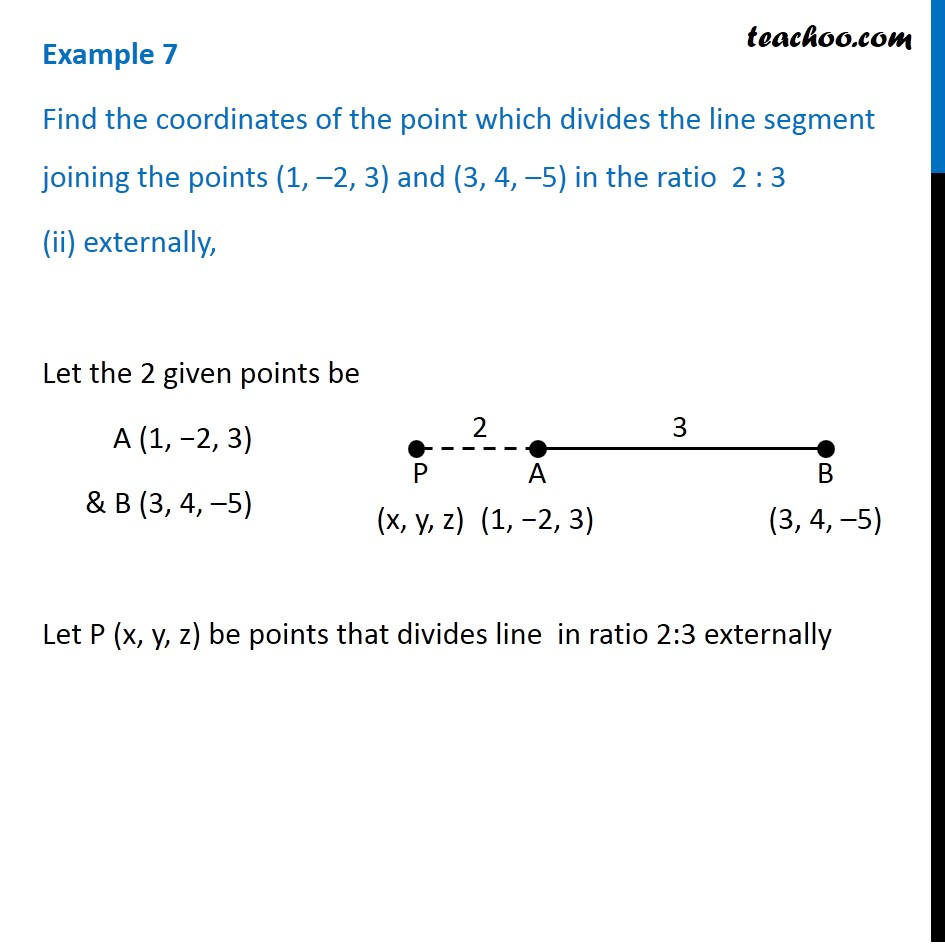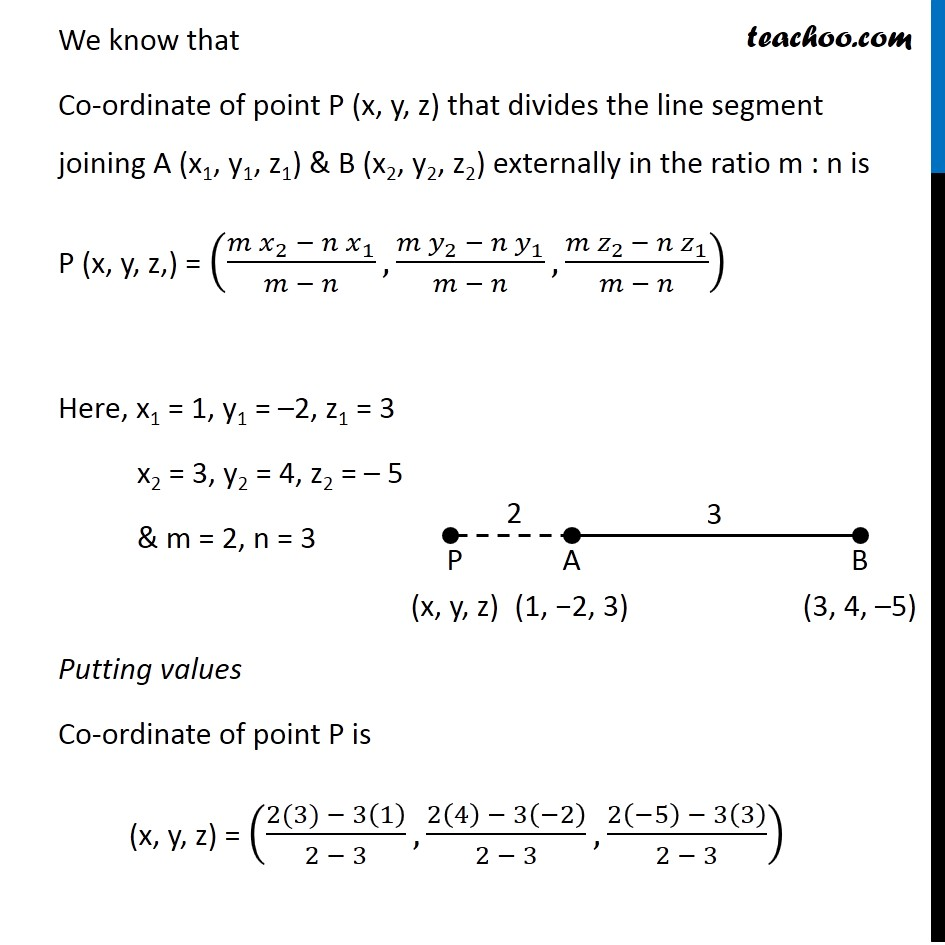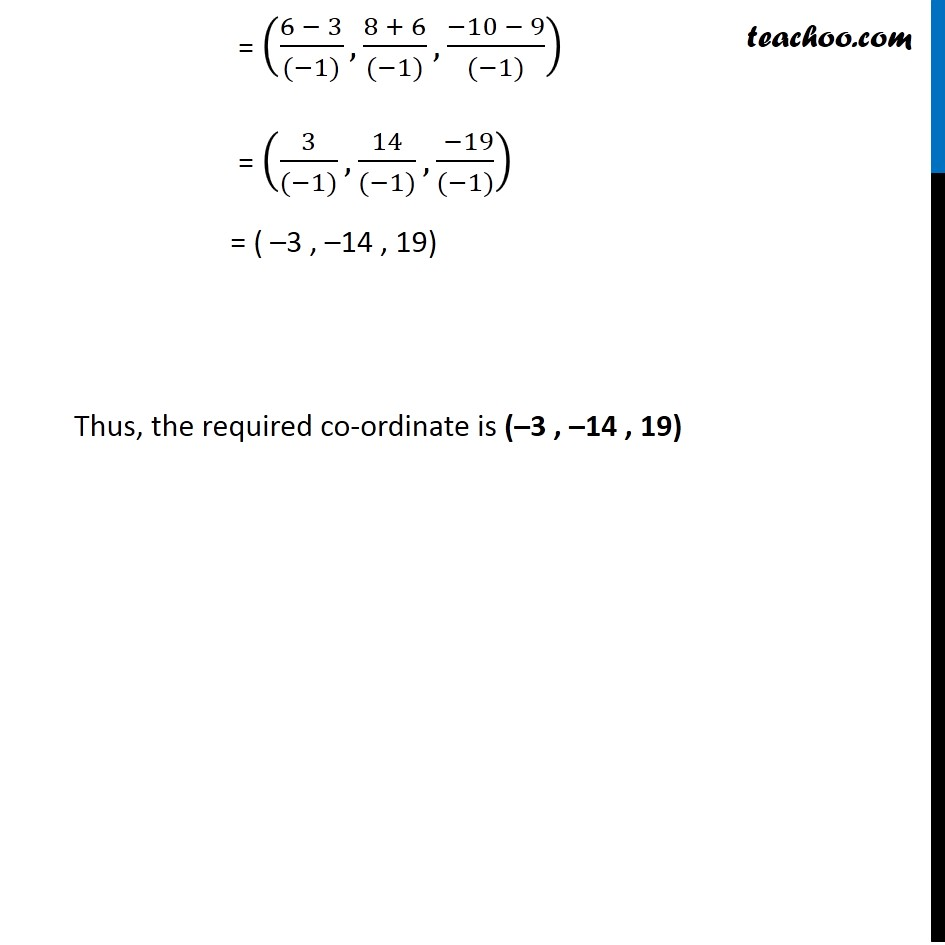Examples

Chapter 12 Class 11 - Intro to Three Dimensional Geometry (Term 2)
Serial order wise### Transcript

Example 7 Find the coordinates of the point which divides the line segment joining the points (1, –2, 3) and (3, 4, –5) in the ratio 2 : 3 (ii) externally, Let the 2 given points be A (1, −2, 3) & B (3, 4, –5) Let P (x, y, z) be points that divides line in ratio 2:3 externally We know that Co-ordinate of point P (x, y, z) that divides the line segment joining A (x1, y1, z1) & B (x2, y2, z2) externally in the ratio m : n is P (x, y, z,) = ((〖𝑚 𝑥〗_2 −〖 𝑛 𝑥〗_1)/(𝑚 − 𝑛),(〖𝑚 𝑦〗_2 −〖 𝑛 𝑦〗_1)/(𝑚 − 𝑛),(〖𝑚 𝑧〗_(2 )−〖 𝑛 𝑧〗_1)/(𝑚 − 𝑛)) Here, x1 = 1, y1 = –2, z1 = 3 x2 = 3, y2 = 4, z2 = – 5 & m = 2, n = 3 Putting values Co-ordinate of point P is (x, y, z) = ((2(3) − 3(1))/(2 − 3),(2(4) − 3(−2))/(2 − 3),(2(−5) − 3(3))/(2 − 3)) = ((6 − 3)/((−1)),(8 + 6)/((−1)),(−10 − 9)/((−1))) = (3/((−1)),14/((−1)),( −19)/((−1))) = ( –3 , –14 , 19) Thus, the required co-ordinate is (–3 , –14 , 19)